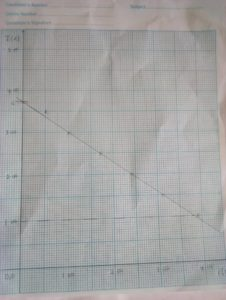NABTEB GCE 2018 PHYSICS (PRACTICAL) QUESTIONS And ANSWERS/EXPO/DUBS/RUNZ

NABTEB GCE 2018 PHYSICS (PRACTICAL) QUESTIONS And ANSWERS/EXPO/DUBS/RUNZ

NABTEB GCE Physics Practical Solution Questions and Answer – MAY/JUNE 2018 Expo Runz,NABTEB GCE 2018 Physics Practical Questions and Answers, NABTEB GCE 2018/2019 ALT physics Practical Answers Expo/Runz,NABTEB GCE 2018 Physicspractical Dubs/Chokes.

NABTEB PHYSICS PRACTICAL SOLUTIONS

(1a)
COMPLETE THE TABLE WITH THIS:

Under S/N: 1, 2, 3, 4, 5, 6
Under p: 4.2, 5.6, 6.9, 8.1, 9.5, 10.6
Under q: 4.8, 6.9, 8.3, 9.9, 11.1, 12.6
Under P: 16.80, 22.40, 27.6, 32.4, 38.0, 42.4
Under Q: 19.20, 27.60, 33.20, 39.60, 44.40, 50.40

(1av)
GRAPH(1avi)
Slope S = DQ/DP = 46.5 – 20.0/40.0 – 15.5
= 26.5/24.5
= 1.08

(1avii)
-I would avoid error due to parallax when using meter rule
-I would ensure that zero error was noted and corrected on metre rule

(1bi)
Density is the mass of a substance per its unit volume while relative density is the ratio of the density of that substance to a certain temperature and the density of water at the same temperature or some other temperature used as a reference.

(1bii)
Given V = 1.5×10^5m^3
m = 3.0×10^-2kg
.:. Density, P = m/v = 3.0 x 10^-2/1.5 x 10^-3
= 2.0 x10^3kgm^-3

(1biii)
PLhL = Pwhw
Where PL is density of liquid = ?
Pw is density of water = 1.0g/cm3
hL is the height of liquid = 15cm
hw is height of water = 10.ocm
PL x 15 = 1 x 10.o
PL = 1 x 10/15
PL = 10/15 = 0.67g/cm3

==============================================

(3a)
COMPLETE THE TABLE WITH THIS and add extra one column:

Under S/N: 1,2,3,4,5,6
Under I: 1.00, 1.50, 2.00, 2.50, 3.00, 3.50
Under V: 3.90, 3.10, 2.40, 1.70, 1.00, 0.50
Under R = V/I: 3.900, 2.067. 1.200, 0.680, 0.333, 0.143

(3av)
GRAPH(3avi)
Slope, S = DI/DV = 3.75-1.00/3.80-0.00
= 2.75/3.80
= 0.724

Intercept C = 3.75

(3avii)
-I would avoid error due to parallax when using the ammeter
-I would ensure tight terminals

(3bi)
Ohm’s law states that the current through a metallic conductor between two points is directly proportional to the potential difference across the two points provided that temperature and other physical condition remains constant.

(3bii)
As the temperature of a metallic conductor increases, the kinetic energy of the electrons of the conductor also increases ,due to which more obstruction is offered to the flowing electrons and hence the current.Therefore,as more obstruction is offered to the flowing electrons, the resistance is increased.

(3biii)
Given E = 3.0V
r = 0.5 ohms
R = 4 ohms
E = I(R+r)
I = E/R+r
= 3.0/4+0.5
= 0.67A

Potential difference = E – IR
= 3.0 – 0.67 x 4
= 3.0 – 2.68
= 0.32V

==-==-=-=–=-=Completed=-=-=-=–=-=